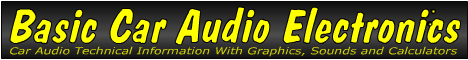Email   Home Page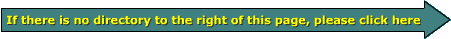x

 Web www.bcae1.com www.bcot1.com
Use F11 to go to full screen
viewing if using Google
Translate.

 If you have a problem with some graphics showing up as large open spaces, empty or black boxes, right-click the link in the directory for the page you're viewing and open the page in a new tab. This will resolve most/all of those issues. Setting the font size at 1 step larger than the default will also help if you want to view the site in it's original format. ^^^^^^^^^^^^^^^^^^^^^^^^^^^ Please Re-Read ^^^^^^^^^^^^^^^^^^^^^^^^^^^

Potentiometers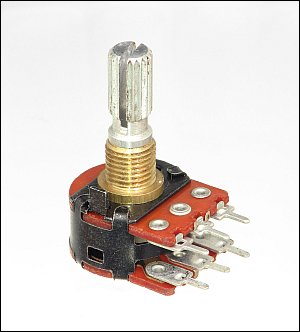There are many instances where only a portion of an output voltage from a signal source is needed. If we allowed the full output voltage from a home CD player to be driven into the input of an amplifier, the amplifier would play at or near full power at all times. This would become quite annoying in a very short period of time. To reduce the overall volume, we need to allow only a fraction of the full signal through to the amplifier. To control the level of the signal, we use a potentiometer. A potentiometer (also know as a 'pot') is a resistor with a movable tap. Potentiometers can be used to allow a change in the resistance in a circuit or as a variable voltage divider (in the case of a volume control). If you have a rotary volume control that doesn't click when rotated, it is (more than likely) a potentiometer being used as a variable voltage divider. If your volume control clicks and steps the volume up or down with each click, it's probably a rotary encoder (a switch), not a potentiometer. A potentiometer generally has 3 terminals. 2 of the terminals are connected (internally) to the opposite ends of a resistive element. The 3rd terminal is called the wiper. It is generally located in-between the other 2 terminals. The wiper is a contact (actually, generally several very small contacts) that makes contact along the resistive element. The diagram below shows the schematic symbol for a potentiometer.

The following diagram shows how the schematic symbol relates to the drawing of the potentiometer.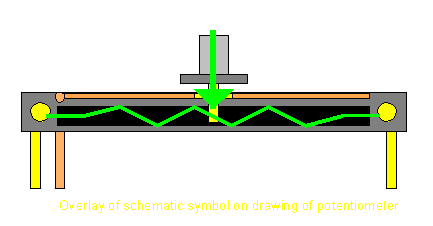This is the inside of the potentiometer shown at the top of the page. The back has been removed and placed next to rest of the potentiometer. The left side shows what the resistive element looks like. It's the wider black area. The inner ring with the line that goes to the center terminal is a non-resistive conductor that passes the signal/voltage from the wiper to the center terminal.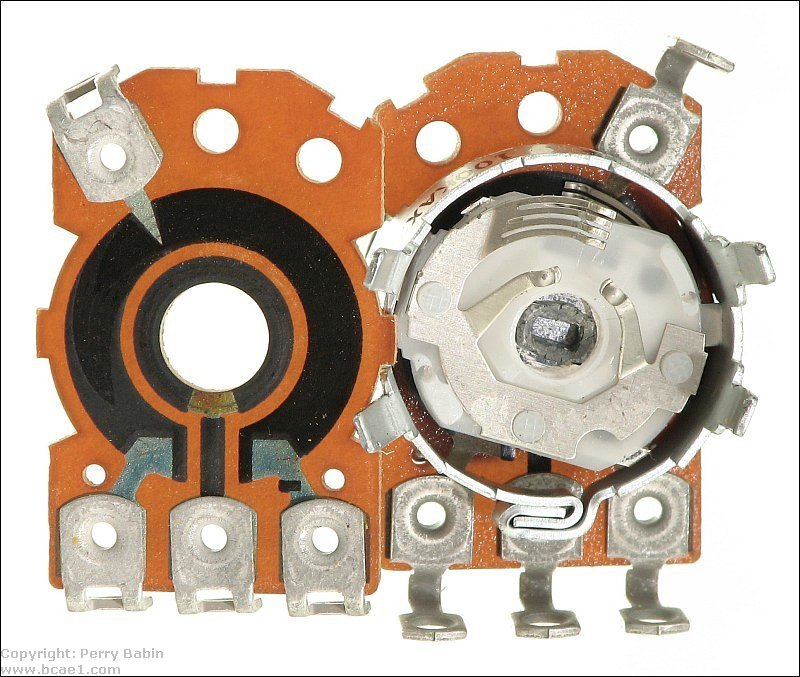Below is a close-up photo of the wiper mechanism. The four parallel contacts at the top make contact with the resistive element. The two contacts below those make contact with the inner ring. If you look 'behind' the white insulator, you can see the wiper contacts for the other half of the potentiometer touching the resistive element.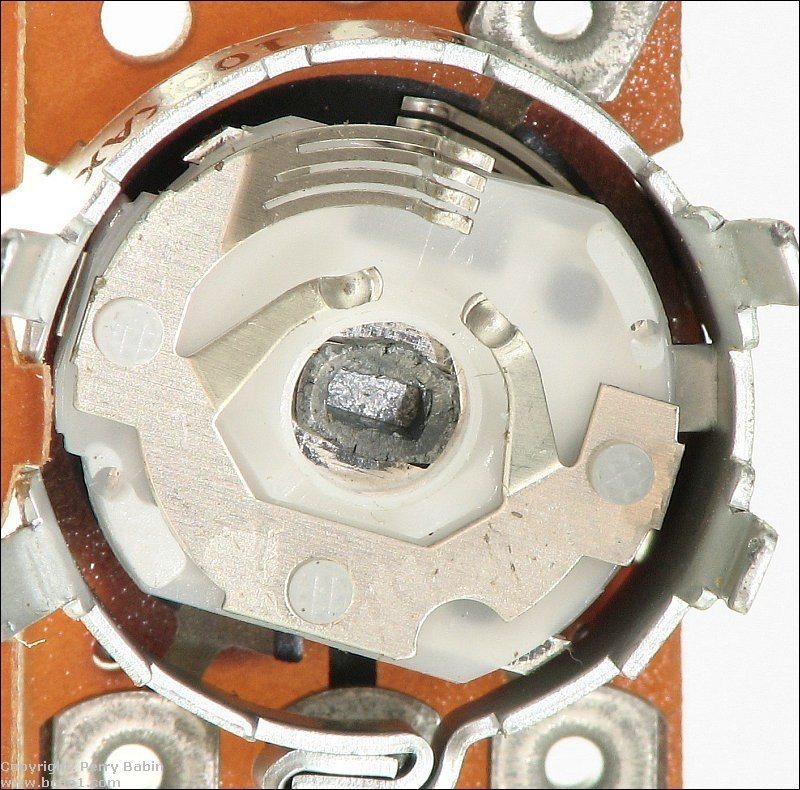Voltage Dividers

A Potentiometer as a Variable Voltage Divider:
We covered voltage dividers on the resistors page. A pot connected as it is in the following diagram will act to divide the voltage like the 2 individual resistors. In the diagram below, you can see that the linear taper potentiometer is in the middle of its range of travel. You can also see that 12 volts is applied to terminals 'A' and 'B' are connected to the 12 volt battery. This means that the output from the slider will be 6 volts.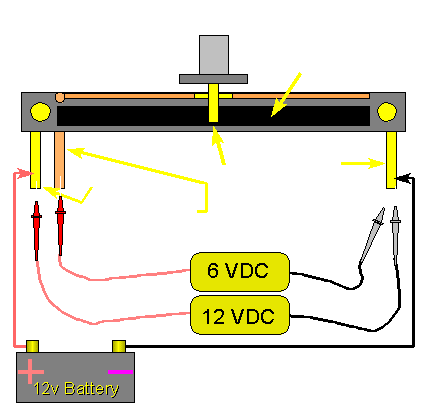This means that the resistance of the resistive element increases in direct proportion to the distance traveled along the resistive element. In the middle of travel, the resistance from the sliding terminal to either of the other terminals is half of the total resistance.

The output is simply the voltage at the point where the wiper contacts the resistive element.

Resistance Taper:
In the previous paragraph, I mentioned that the potentiometer had a 'linear' taper. These are general purpose potentiometers and may be used for controlling DC voltage (as it did in the diagram) or to control the levels of the individual bands on an equalizer. For volume controls you need to use a potentiometer with a 'log' (short for logarithmic) taper. This is because the human auditory system works logarithmically. If we'd use a linear taper pot for a volume control, the low end of the volume control's range would be 'touchy'. What I mean to say is that the volume would seem to increase really fast at the bottom end of the volume control's range and would require a lot of travel at the upper end of the volume control's range. As you can see by the graph below, the resistance of the linear pot (red line) at 50% of its travel is 50% of its resistance. The log pot's resistance is far less than 50% at 50% of its travel. You have to go to about 85% of its travel to reach the midpoint of its resistance (500 ohms for a 1000 ohm pot).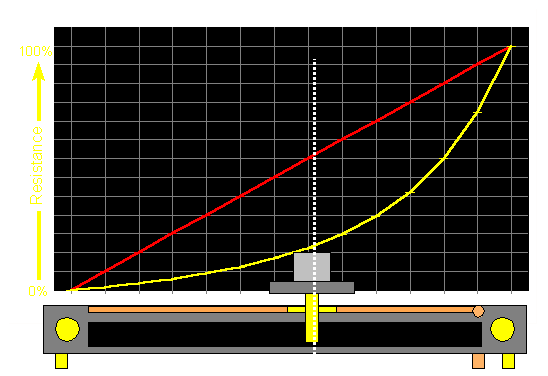In the next diagram, you can see that the slider has been moved toward the positive terminal. This means that the output voltage will be higher than 6 volts. In this case the output voltage is 7.5 volts DC. If the slider was moved closer to the negative terminal, the voltage would be lower.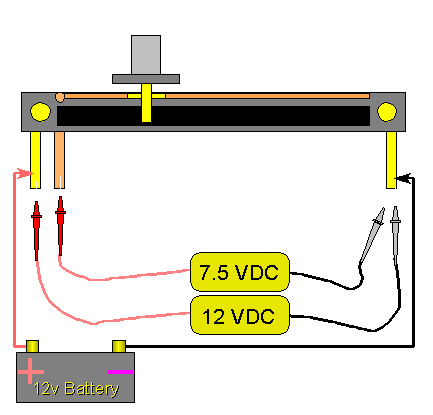Output with AC:
If the pot was connected to an AC signal generator (i.e. sine wave generator) instead of a battery, the voltage on the output terminal would be ~63% of the input voltage from the generator. If the input signal was 12 volts AC, the output would be 7.5 volts AC. The following diagram shows the input and the output voltages graphically with both AC and DC input voltages. It is based on the same slider position as was used in the previous diagram.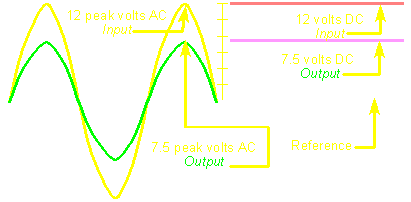Supply Voltage? Volts Pot's Total Resistance? Ohms Position (0-100%)? % Wiper Voltage (Linear): Volts Wiper Voltage (Logarithmic): Volts Pot's Power Dissipation: Watts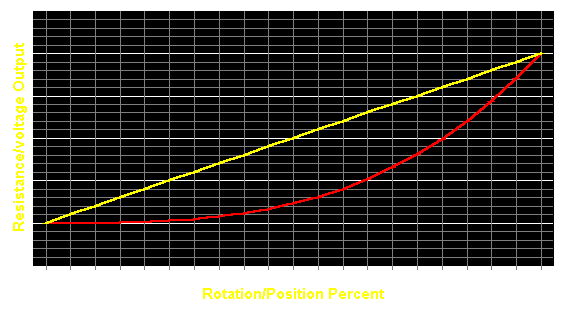Rotary Potentiometers:
The following diagram shows a rotary potentiometer at 50% and ~63% of its range of travel. These positions would correspond to the positions of the slider pot in the previous diagram. This is a single turn potentiometer which means that it can travel its entire range within 1 complete revolution if its shaft (knob). Actually, a single turn pot will only travel through about 270� of a complete revolution. There are other potentiometers that have some sort of mechanism to increase the number of times the shaft must be turned to travel through its entire range of motion. This is generally done with gears but I've seen it done in a planetary gear configuration using ball bearings. The multi-turn potentiometers are used to make it easier to precisely set the output level.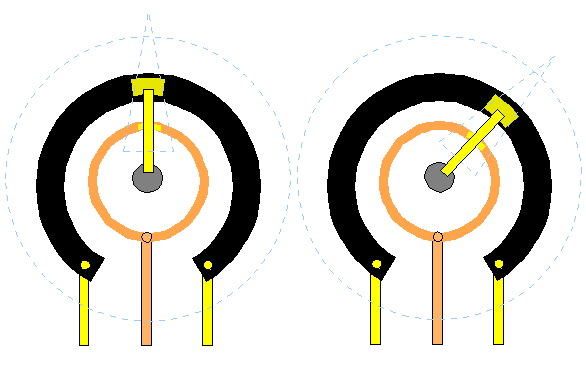Power Ratings:
Potentiometers have power ratings just as resistors do. When the potentiometer are used for preamp level audio and such, power dissipation isn't really a problem. If you are using the potentiometer to control a signal with significant current flow, you'd have to calculate the power dissipation across its resistive element and use a potentiometer of a sufficient power rating. Refer to the Ohm's Law page for the appropriate formula.

In the picture below, at the top is a multi-section potentiometer used in older head units. Below, various styles of printed circuit board potentiometers.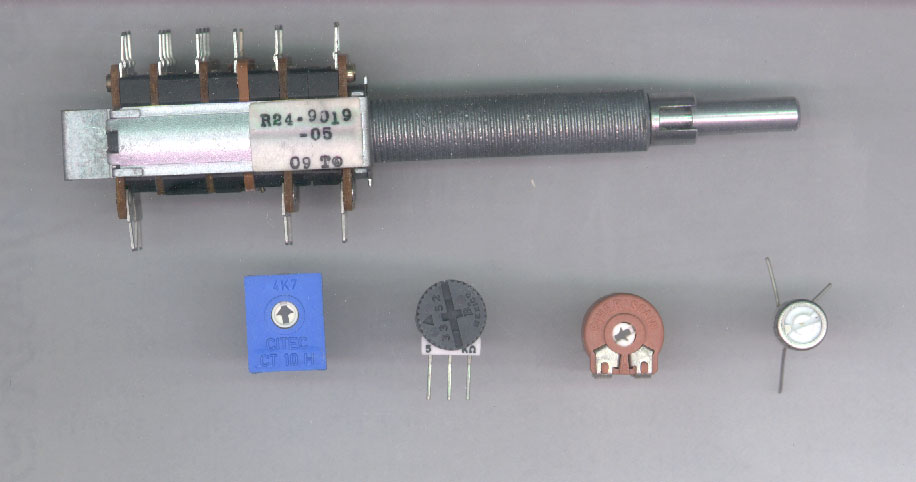RCA/Preamp Level Controllers

The images below show how to wire a volume control to be inserted into the preamp line. If noise is a problem when you touch the metal shaft, ground the metal part of the potentiometer. A ring terminal around the threaded bushing is probably the best way to ground it. A potentiometer specifically designed for use as a volume control (a pot with a 'logarithmic' taper) will give the most linear volume control. The Radio Shack part # 271-1732 is a good choice. To connect between two components (head units and amplifiers or head units and crossovers), you would use RCA connectors for the input and output. For those using an MP3 player (iPod, iPad, cell phone...), you would use whatever connector is needed on the input to fit the MP3 player.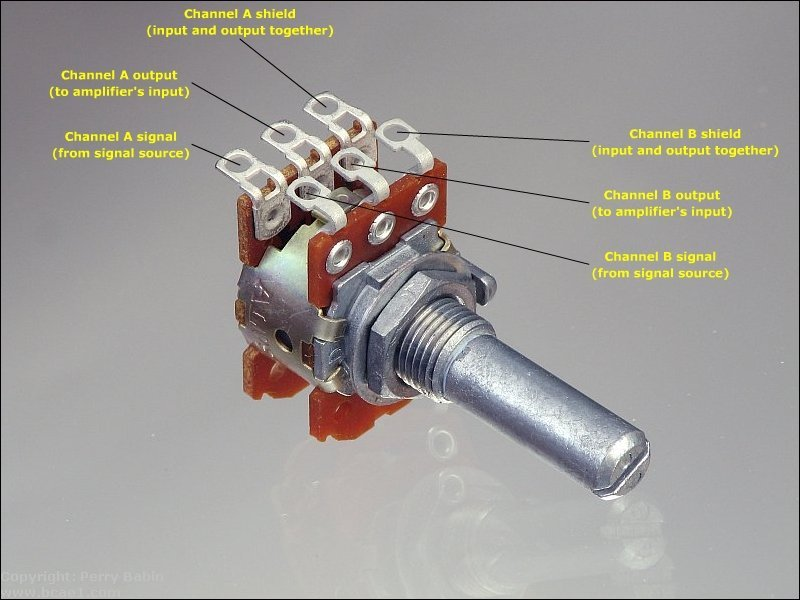Below, you can see one half of the potentiometer connected to the shielded cable. It doesn't matter which half of the pot you use for left and right. The two halves are identical. In this photo, you can see which shielded cable each of the red wires comes out of. The top black cable would go to the amplifiers or crossover. The bottom black cable would go to the signal source (head unit or MP3 player). You can see how the shields for both cable (input and output for the channel) are tied together.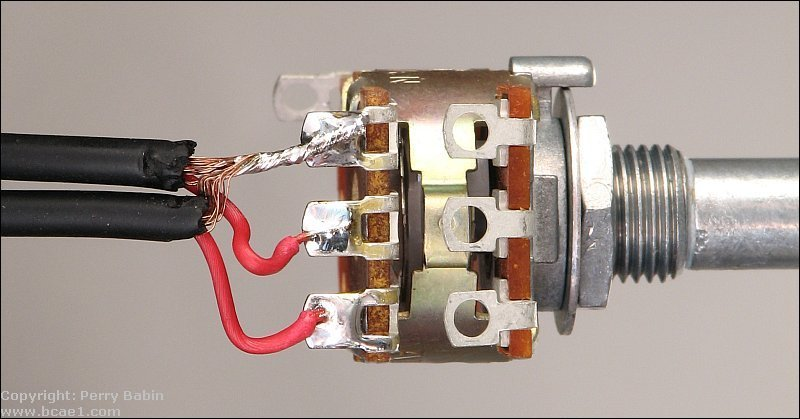Above, notice how the shields are tightly twisted together except for a short length near where they enter the insulated part of the cable. This is done for a reason. If you tightly twist the shield all of the way to the insulator, the heat will be efficiently transferred through the braided shield to the insulation for the center conductor. This can cause strands of the shield to melt into the insulation which will allow either constant or intermittent contact with the center conductor. When the shield contacts the center conductor, the signal will be shorted to ground and no audio will pass to the next component.

Click HERE to make the demo fill this window.

 You should remember: 1.The voltage on the wiper terminal of a linear taper potentiometer has a voltage which is directly proportional to its physical position along its resistive element.

Click HERE to visit a friend's new car audio tech site.

```

```